# CALCULATION HACKS WHICH WILL ERASE YOUR HATRED TOWARDS MATHS !!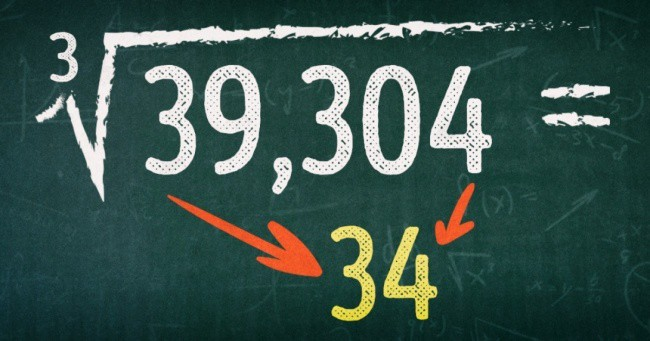Who likes doing maths? I am sure there will be less then a few hands going up. Not everyone has a way with maths. But, here are some fantastic and straightforward calculation hacks which will make maths your cup of tea.

#### 1. TAKING OUT THE PERCENTAGE OF A NUMBER IS NOT A BIG DEAL NOW.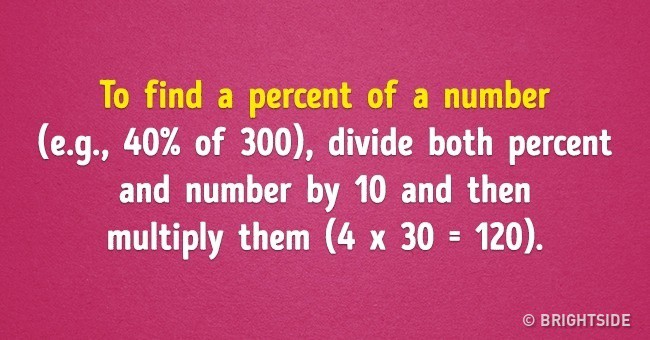#### 2.  EASY SQUARE CALCULATION

Note- This trick is valid for all the numbers having five at one’s place.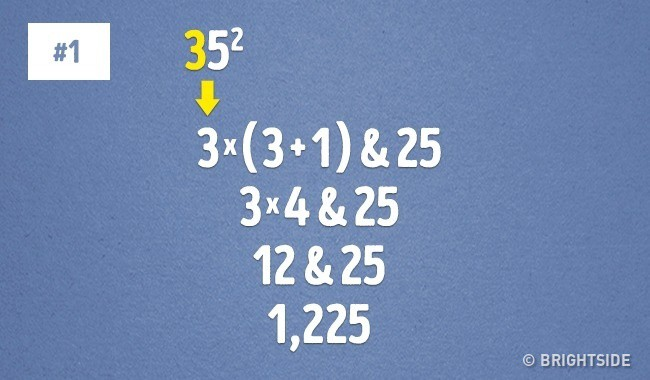#### 3. EASIEST TABLE OF 9, AS YET!#### 4. MULTIPLY BY 5 IN JUST A FEW SECONDS, NOW!

First, divide the number by 2 and see if it’s a whole number. If it is a whole number, then add 0 at the end. But, if it’s not a whole number, then ignore the decimal and add five at the end.#### 5. QUICK MULTIPLICATION BY 11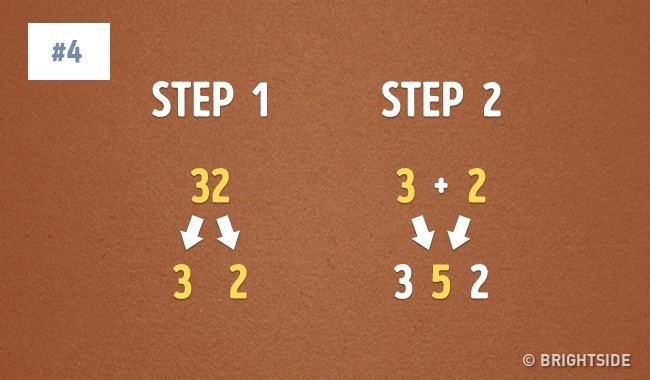#### 6. MULTIPLICATION BY 4 MADE EASY!!

Multiply the number by 2 and, repeat. Now add. Tadaa!#### 7. EASY-BIZZY WAY TO CALCULATE TIPS

Need to find what is the 15% of the order that you’ve to leave as a tip? Watch this.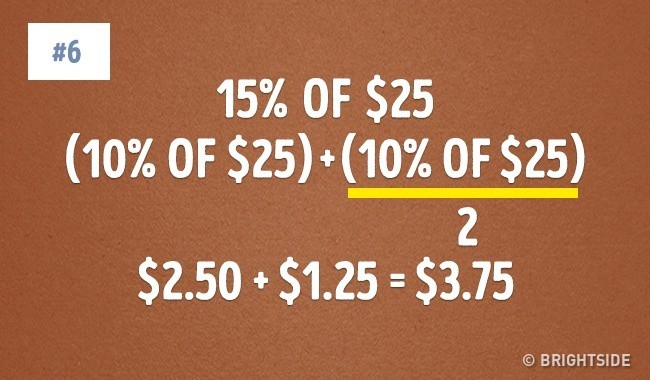#### 8. ANOTHER MULTIPLICATION TRICK

If there is an even number, just regroup the numbers to reach to the answer.#### 9. DIVISION BY 5#### 10. SUBTRACTION BY 1000

Subtract the digits by 9 and the last figure by 10. And, here is your answer.#### 11. CONVERSION FROM CELSIUS TO FAHRENHEIT AND BACK.#### 12. GET A CUBE ROOT INSTANTLY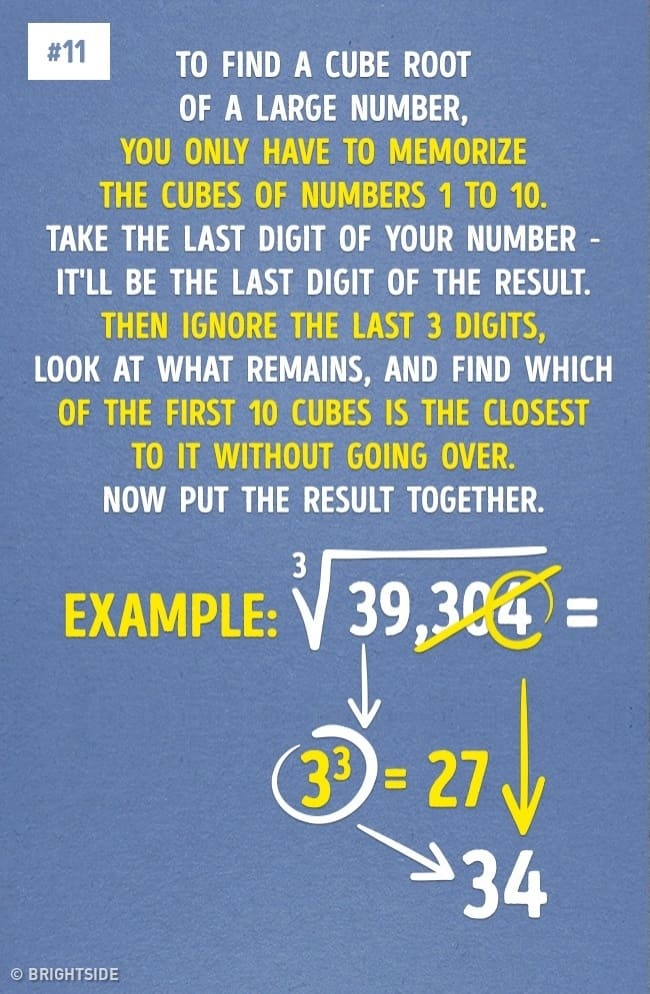Popular
18 pictures showing the day to day struggles of a woman's life.